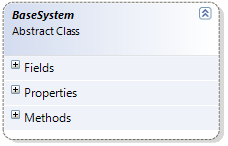Scientific Research Software

Resource for Scientific Researchers and Engineering Software Developers.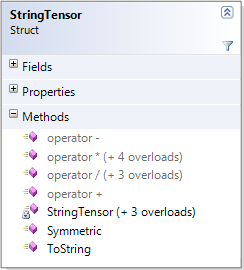Libraries for Engineering Software Developers

Unique libraries for mathematical, physical and engineering software developing.

• 100% source code.
• Strongly structured class hierarchies.
• Easy to understand and use.
• Easy to introduce your specific features.
• Use with minimal code writing.

ANALYTICS library: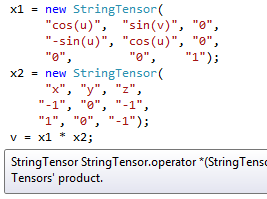• Using analytical calculations in program code.
• Parsing and manipulating analytical expression in program code.
• Input analytical expression as string data with syntax checking.
• Making analytical calculations with any specific data.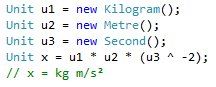PHYSICS library:

• Using units of measurement and physical quantities in program code.
• Making unit conversions in program code with dimension checking.
• Manipulating measured data in program code with overloaded operators.
• Input data with associated units of measurement.

Software for Engineering and Mathematical modeling

REGIONS Multi-Physics – boundary value problem solver. The application is based on unique analytical method – Fictitious Canonic Region method.

REGIONS Gamma-Gamma – simulator of gamma quantum interaction with continua based on the Monte-Carlo statistical modeling.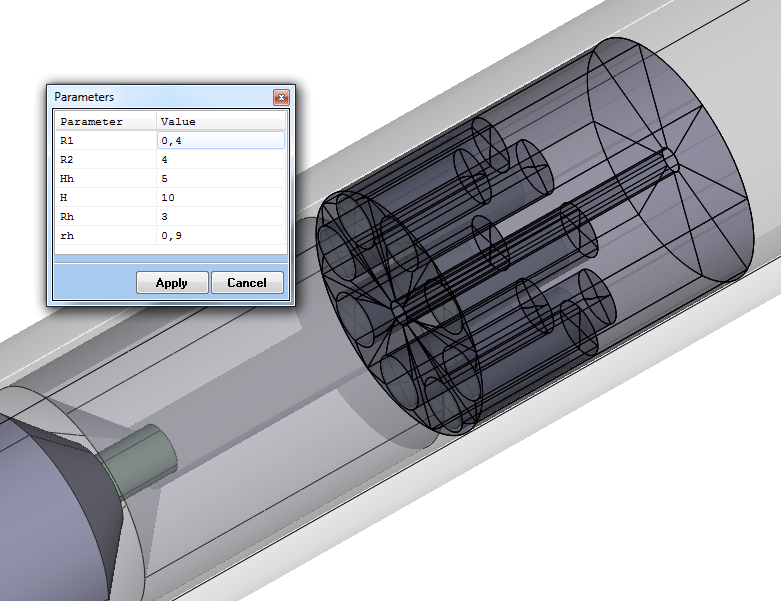All modeling applications use unique, totally analytical and parametric geometry modeler. Using parametric geometry models is the core feature for researchers. This feature provides ‘parametric investigation’ of the solving problem – understand how geometry properties influence the solution results.

Software for Well Log Data Processing

WLD CAD – well logging data processor.  The program is based on the ANALYTICS technology and allows calculating result data by any (nonstandard) formula.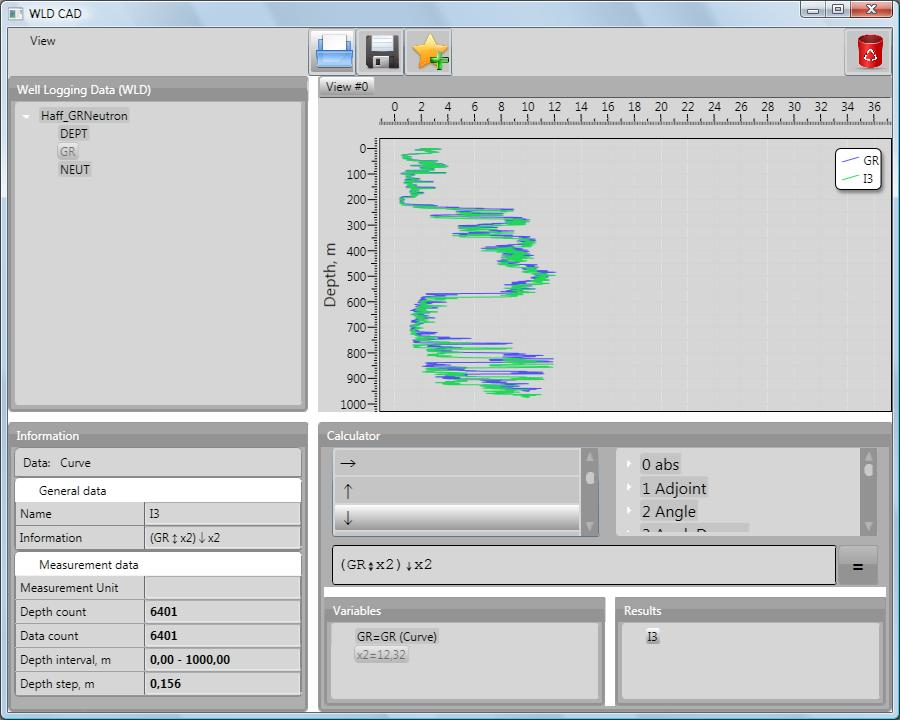WLD CAD analytical library contains many predefined operations with well logging data:

• standard algebraic, transcendental and special functions (trigonometric, hyperbolic, logarithmic, exponential and so on);
• standard mathematical operators (addition, subtraction, multiplication and so on);
• special operations for well logging signals (fast Fourier transform, maximum, minimum, unit conversion and so on);
• special operations for depth management (shift, extension, range extraction and so on).

Using unique ANALYTICS technology allows combine all operations in any set and order – and so, get almost any processing algorithm.

Services